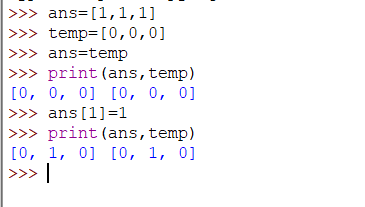# Closing the tweets

n, k = map(int, input().split())
ans = []
temp = []
for t in range(n):
ans.append(0)
temp.append(0)
for i in range(k):
s = input()
if s==“CLOSEALL”:
ans = temp
print(sum(ans))
else:
s1,num = s.split()
num = int(num)
if(ans[num-1]==0):
ans[num-1] = ans[num-1]+1
else:
ans[num-1] = ans[num-1]-1

``````    print(sum(ans))
``````

i am not getting where i did wrong. plz help me, suggestions are welcome!

1 Like

The entire code was perfect, but you have committed a minute mistake, which is as follows:
On ans = temp, you don’t actually have two lists. The assignment just copies the reference to the list, not the actual list, so both ans and temp refer to the same list after the assignment.
I’ve taken a piece of code for explaining it more clearlyI’ve also taken a sample test case to show where you’re going wrong. In the same test case At last, I’ve taken CLOSEALL again where temp is no longer referring to original array *n, in fact it’s referring to the same ans list.

So you have to change your code a bit, as follows:

Hope it’s useful.#happy coding